Topics in Demand and Supply Analysis | IFT World
IFT Notes for Level I CFA® Program
IFT Notes for Level I CFA® Program

# Part 2

### 5.     Income Elasticity of Demand, Cross-Price Elasticity of Demand

Income elasticity of demand is the percentage change in the quantity demanded divided by a percentage change in income, all else constant. It measures how sensitive the quantity demanded is to changes in income. It is expressed as: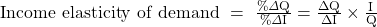If income elasticity of demand is 0.6, then it means that for every 1% increase in income, the quantity demanded will increase by 0.6%. While own-price elasticity is usually negative, income elasticity of demand can be positive, negative, or zero.

Positive income elasticity means that as income increases, quantity demanded also increases. Negative income elasticity means that as income increases, quantity demanded for these goods decreases.

Based on income elasticity, goods can be categorized as normal or inferior:

• Normal good: Income elasticity is positive. That is, as income rises, quantity demanded (consumption of the good) also rises.
• Inferior good: Income elasticity is negative. That is, as income rises, people buy less of these goods. For example, bicycles in South Asia and fast food in US.

As discussed earlier a change in any variable other than own price would cause the demand curve to shift. Therefore, a change in income will cause a shift in the demand curve.

### Cross-Price Elasticity of Demand

Cross-price elasticity of demand measures how sensitive the quantity demanded of a good, X, is to changes in the price of another related good, Y, all else constant. The equation for the cross-price elasticity of demand is similar to the own-price elasticity of demand except that the denominator uses the price of another good, Y. It is expressed as: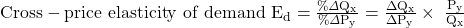Substitutes: Two goods are substitutes if one can be used instead of the other. The cross-price elasticity of demand is positive for substitute goods.  An increase in the price of a substitute good would increase the quantity demanded of the subject good.

Complements: Two goods are complements if they are used together. The cross-price elasticity of demand is negative for complement goods.  An increase in the price of a complement good would decrease the quantity demanded of the subject good. Example: cereal and milk, and petrol and cars.

Example

A consumer’s weekly demand for coffee is given by the following demand function: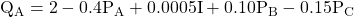Assume the price of coffee is PA = 10, the price of tea is PB = 55, the price of lemon water is PC = 10, and income is I = 2,000. Given this data, calculate the following:

1. Own-price elasticity of demand for A.
2. Income elasticity of demand for A.
3. Cross-price elasticity of demand of A against price of B.
4. Are A and B substitutes or complements?
5. Cross-price elasticity of demand of A against price of C.
6. Are A and C substitutes or complements?

Solution:

1. First, calculate the value of Q by plugging in the values for PA = 10, PB = 55, PC = 10, I = 2,000 in the demand function.
2.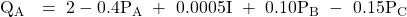1.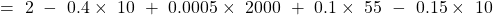2.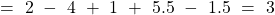3.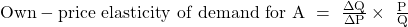4.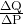is the own-price elasticity coefficient, which is -0.4 from the equation.
5. So, own-price elasticity of demand =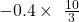= – 1.333
1. Income elasticity of demand for A =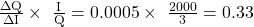2. Cross-price elasticity of demand of A against price of B =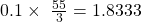3. A and B are substitutes because as the price of B goes up, the quantity demanded of A goes up. A positive relationship indicates the products are substitutes.
4. Cross-price elasticity of demand of A against price of C =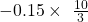= -0.5
5. A negative relationship between the price of C and the quantity demanded of A means the two products are complements.

### 6.     Substitution and Income Effects; Normal Goods, Inferior Goods and Special Cases

The law of demand states that when a good’s own-price falls, its quantity demanded increases, all else equal. Let us take an example of a hypothetical two-goods economy consisting of bread and milk to understand the two reasons why quantity demanded rises when price falls. Assume the price of bread decreases.  Bread is now cheaper relative to milk, so consumers buy more bread. This is called the substitution effect. A lower price of bread means the consumer has greater buying power, which implies that the real income has increased. The change in income will impact how much bread consumers buy. This is called the income effect.

### Normal and Inferior Goods

The table below summarizes the substitution and income effects of a price decrease for normal and inferior goods:

 Substitution Effect Income Effect Normal good Buy more because when the good’s price decreases, it is relatively cheaper than its substitutes. Buy more because there is an increase in real income that increases the consumption. Inferior good Buy more because when the good’s price decreases, it is relatively cheaper than its substitutes. Buy less because the increase in real income causes the consumer to buy less of the inferior good.

Giffen goods:

A Giffen good is an extreme case of an inferior good where the income effect dominates the substitution effect. In this case, a decrease in price causes a decrease in quantity demanded which implies a positively sloped demand curve. Hence Giffen goods are an exception to the law of demand. The curriculum identifies rice in rural China as a possible Giffen good. When the price of rice decreased, consumers in rural China with very low incomes decreased their intake of rice and switched to alternatives such as meat that provided more calories.

Veblen goods

Like Giffen goods, Veblen goods also have an upward sloping demand curve and hence they also violate the law of demand. However, the similarity ends there.  The characteristics of Veblen goods are described below:

• Veblen goods are status goods; an increase in price increases the value to some consumers and therefore their quantity demanded increases.
• Veblen goods are based on the concept of conspicuous consumption. This means that consumers derive utility from the fact that others regard them as someone who consumes an expensive good. As the price of such products increase, consumers will be inclined to purchase more to flaunt their affluence. For example, a Bugatti Veyron car, a yacht, or a private island.

LIVE WEEKEND CLASSES *For 2024 Exam candidates*
This is default text for notification bar